Fixed-Point Code Generation for Prediction of SVM

This example shows how to generate fixed-point C/C++ code for the prediction of a support vector machine (SVM) model. Compared to the general C/C++ code generation workflow, fixed-point code generation requires an additional step that defines the fixed-point data types of the variables required for prediction. Create a fixed-point data type structure by using generateLearnerDataTypeFcn, and use the structure as an input argument of loadLearnerForCoder in an entry-point function. You can also optimize the fixed-point data types before generating code.

This flow chart shows the fixed-point code generation workflow.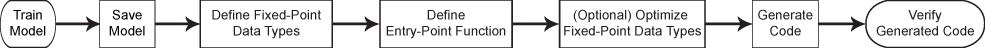1. Train an SVM model.

2. Save the trained model by using saveLearnerForCoder.

3. Define the fixed-point data types of the variables required for prediction by using the data type function generated by generateLearnerDataTypeFcn.

4. Define an entry-point function that loads the model by using both loadLearnerForCoder and the structure, and then calls the predict function.

5. (Optional) Optimize the fixed-point data types.

6. Generate fixed-point C/C++ code.

7. Verify the generated code.

Step 5 is an optional step to improve the performance of the generated fixed-point code. To do so, repeat these two steps until you are satisfied with the code performance:

1. Record minimum and maximum values of the variables for prediction by using buildInstrumentedMex (Fixed-Point Designer).

2. View the instrumentation results using showInstrumentationResults (Fixed-Point Designer). Then, tune the fixed-point data types (if necessary) to prevent overflow and underflow, and to improve the precision of the fixed-point code.

In this workflow, you define the fixed-point data types by using the data type function generated from generateLearnerDataTypeFcn. Separating data types of the variables from the algorithm makes testing simpler. You can programmatically toggle data types between floating-point and fixed-point by using the input argument of the data type function. Also, this workflow is compatible with Manual Fixed-Point Conversion Workflow (Fixed-Point Designer).

Preprocess Data

Load the census1994 data set. This data set consists of demographic data from the US Census Bureau used to predict whether an individual makes over \$50,000 a year.

Consider a model that predicts the salary category of employees given their age, working class, education level, capital gain and loss, and number of working hours per week. Extract the variables of interest and save them using a table.

Print a summary of the table.

summary(tbl)
Variables:

age: 32561x1 double

Values:

Min          17
Median       37
Max          90

education_num: 32561x1 double

Values:

Min           1
Median       10
Max          16

capital_gain: 32561x1 double

Values:

Min            0
Median         0
Max        99999

capital_loss: 32561x1 double

Values:

Min            0
Median         0
Max         4356

hours_per_week: 32561x1 double

Values:

Min           1
Median       40
Max          99

The scales of the variables are not consistent. In this case, you can train a model using a standardized data set by specifying the 'Standardize' name-value pair argument of fitcsvm. However, adding the operations for standardization to the fixed-point code can reduce precision and increase memory use. Instead, you can manually standardize the data set, as shown in this example. The example also describes how to check the memory use at the end.

Fixed-point code generation does not support tables or categorical arrays. So, define the predictor data X using a numeric matrix, and define the class labels Y using a logical vector. A logical vector uses memory most efficiently in a binary classification problem.

X = table2array(tbl);

Define the observation weights w.

The memory use of a trained model increases as the number of support vectors in the model increases. To reduce the number of support vectors, you can increase the box constraint when training by using the 'BoxConstraint' name-value pair argument or use a subsampled representative data set for training. Note that increasing the box constraint can lead to longer training times, and using a subsampled data set can reduce the accuracy of the trained model. In this example, you randomly sample 1000 observations from the data set and use the subsampled data for training.

rng('default') % For reproducibility
[X_sampled,idx] = datasample(X,1000,'Replace',false);
Y_sampled = Y(idx);
w_sampled = w(idx);

Find the weighted means and standard deviations by training the model using the 'Weight' and 'Standardize' name-value pair arguments.

tempMdl = fitcsvm(X_sampled,Y_sampled,'Weight',w_sampled,'KernelFunction','gaussian','Standardize',true);
mu = tempMdl.Mu;
sigma = tempMdl.Sigma;

If you do not use the 'Cost', 'Prior', or 'Weight' name-value pair argument for training, then you can find the mean and standard deviation values by using the zscore function.

[standardizedX_sampled,mu,sigma] = zscore(X_sampled);

Standardize the predictor data by using mu and sigma.

standardizedX = (X-mu)./sigma;
standardizedX_sampled = standardizedX(idx,:);

You can use a test data set to validate the trained model and to test an instrumented MEX function. Specify a test data set and standardize the test predictor data by using mu and sigma.

standardizedXTest = (XTest-mu)./sigma;

Train Model

Train a binary SVM classification model.

Mdl = fitcsvm(standardizedX_sampled,Y_sampled,'Weight',w_sampled,'KernelFunction','gaussian');

Mdl is a ClassificationSVM model.

Compute the classification error for the training data set and the test data set.

loss(Mdl,standardizedX_sampled,Y_sampled)
ans = 0.1663
loss(Mdl,standardizedXTest,YTest)
ans = 0.1905

The SVM classifier misclassifies approximately 17% of the training data and 19% of the test data.

Save Model

Save the SVM classification model to the file myMdl.mat by using saveLearnerForCoder.

saveLearnerForCoder(Mdl,'myMdl');

Define Fixed-Point Data Types

Use generateLearnerDataTypeFcn to generate a function that defines the fixed-point data types of the variables required for prediction of the SVM model. Use all available predictor data to obtain realistic ranges for the fixed-point data types.

generateLearnerDataTypeFcn('myMdl',[standardizedX; standardizedXTest])

generateLearnerDataTypeFcn generates the myMdl_datatype function. Display the contents of myMdl_datatype.m by using the type function.

type myMdl_datatype.m
function T = myMdl_datatype(dt)
%MYMDL_DATATYPE Define data types for fixed-point code generation
%
%   T = MYMDL_DATATYPE(DT) returns the data type structure T, which defines
%   data types for the variables required to generate fixed-point C/C++ code
%   for prediction of a machine learning model. Each field of T contains a
%   fixed-point object returned by fi. The input argument dt specifies the
%   DataType property of the fixed-point object. Specify dt as 'Fixed' (default)
%   for fixed-point code generation or specify dt as 'Double' to simulate
%   floating-point behavior of the fixed-point code.
%
%   Use the output structure T as both an input argument of an entry-point
%   function and the second input argument of loadLearnerForCoder within the

%   File: myMdl_datatype.m
%   Statistics and Machine Learning Toolbox Version 12.2 (Release R2021b)
%   Generated by MATLAB, 01-Sep-2021 14:32:31

if nargin < 1
dt = 'Fixed';
end

% Set fixed-point math settings
fm = fimath('RoundingMethod','Floor', ...
'OverflowAction','Wrap', ...
'ProductMode','FullPrecision', ...
'MaxProductWordLength',128, ...
'SumMode','FullPrecision', ...
'MaxSumWordLength',128);

% Data type for predictor data
T.XDataType = fi([],true,16,11,fm,'DataType',dt);

% Data type for output score
T.ScoreDataType = fi([],true,16,14,fm,'DataType',dt);

% Internal variables
% Data type of the squared distance dist = (x-sv)^2 for the Gaussian kernel G(x,sv) = exp(-dist),
% where x is the predictor data for an observation and sv is a support vector
T.InnerProductDataType = fi([],true,16,6,fm,'DataType',dt);

end

Note: If you click the button located in the upper-right section of this example and open the example in MATLAB®, then MATLAB opens the example folder. This folder includes the entry-point function file.

The myMdl_datatype function uses the default word length (16) and proposes the maximum fraction length to avoid overflows, based on the default word length (16) and safety margin (10%) for each variable.

Create a structure T that defines the fixed-point data types by using myMdl_datatype.

T = myMdl_datatype('Fixed')
T = struct with fields:
XDataType: [0x0 embedded.fi]
ScoreDataType: [0x0 embedded.fi]
InnerProductDataType: [0x0 embedded.fi]

The structure T includes the fields for the named and internal variables required to run the predict function. Each field contains a fixed-point object, returned by fi (Fixed-Point Designer). For example, display the fixed-point data type properties of the predictor data.

T.XDataType
ans =

[]

DataTypeMode: Fixed-point: binary point scaling
Signedness: Signed
WordLength: 16
FractionLength: 11

RoundingMethod: Floor
OverflowAction: Wrap
ProductMode: FullPrecision
MaxProductWordLength: 128
SumMode: FullPrecision
MaxSumWordLength: 128

For more details about the generated function and the structure, see Data Type Function.

Define Entry-Point Function

Define an entry-point function named myFixedPointPredict that does the following:

• Accept the predictor data X and the fixed-point data type structure T.

• Load a fixed-point version of a trained SVM classification model by using both loadLearnerForCoder and the structure T.

• Predict labels and scores using the loaded model.

function [label,score] = myFixedPointPredict(X,T) %#codegen
[label,score] = predict(Mdl,X);
end

(Optional) Optimize Fixed-Point Data Types

Optimize the fixed-point data types by using buildInstrumentedMex and showInstrumentationResults. Record minimum and maximum values of all named and internal variables for prediction by using buildInstrumentedMex. View the instrumentation results using showInstrumentationResults; then, based on the results, tune the fixed-point data type properties of the variables.

Specify Input Argument Types of Entry-Point Function

Specify the input argument types of myFixedPointPredict using a 2-by-1 cell array.

ARGS = cell(2,1);

The first input argument is the predictor data. The XDataType field of the structure T specifies the fixed-point data type of the predictor data. Convert X to the type specified in T.XDataType by using the cast (Fixed-Point Designer) function.

X_fx = cast(standardizedX,'like',T.XDataType);

The test data set does not have the same size as the training data set. Specify ARGS{1} by using coder.typeof (MATLAB Coder) so that the MEX function can take variable-size inputs.

ARGS{1} = coder.typeof(X_fx,size(standardizedX),[1,0]);

The second input argument is the structure T, which must be a compile-time constant. Use coder.Constant (MATLAB Coder) to specify T as a constant during code generation.

ARGS{2} = coder.Constant(T);

Create Instrumented MEX Function

Create an instrumented MEX function by using buildInstrumentedMex (Fixed-Point Designer).

• Specify the input argument types of the entry-point function by using the -args option.

• Specify the MEX function name by using the -o option.

• Compute a histogram by using the -histogram option.

• Allow full code generation support by using the -coder option.

buildInstrumentedMex myFixedPointPredict -args ARGS -o myFixedPointPredict_instrumented -histogram -coder
Code generation successful.

Test Instrumented MEX Function

Run the instrumented MEX function to record instrumentation results.

[labels_fx1,scores_fx1] = myFixedPointPredict_instrumented(X_fx,T);

You can run the instrumented MEX function multiple times to record results from various test data sets. Run the instrumented MEX function using standardizedXTest.

Xtest_fx = cast(standardizedXTest,'like',T.XDataType);
[labels_fx1_test,scores_fx1_test] = myFixedPointPredict_instrumented(Xtest_fx,T);

View Results of Instrumented MEX Function

Call showInstrumentationResults (Fixed-Point Designer) to open a report containing the instrumentation results. View the simulation minimum and maximum values, proposed fraction length, percent of current range, and whole number status.

showInstrumentationResults('myFixedPointPredict_instrumented')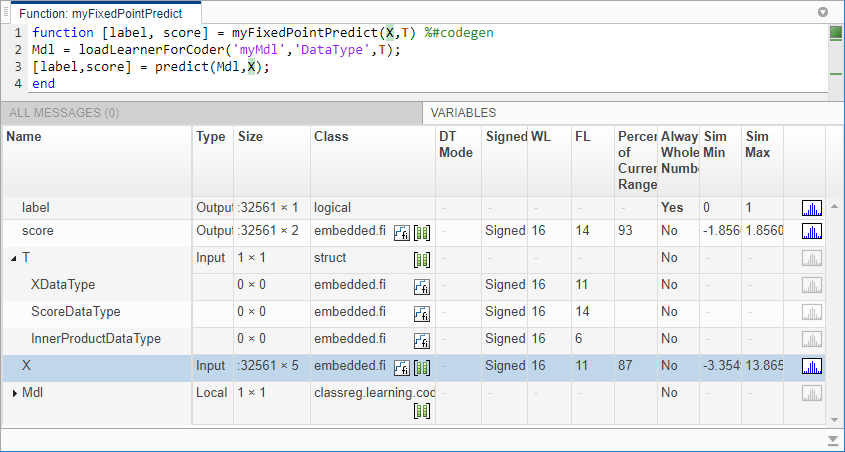The proposed word lengths and fraction lengths in X are the same as those in XDataType in the structure T.

View the histogram for a variable by clicking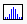on the Variables tab.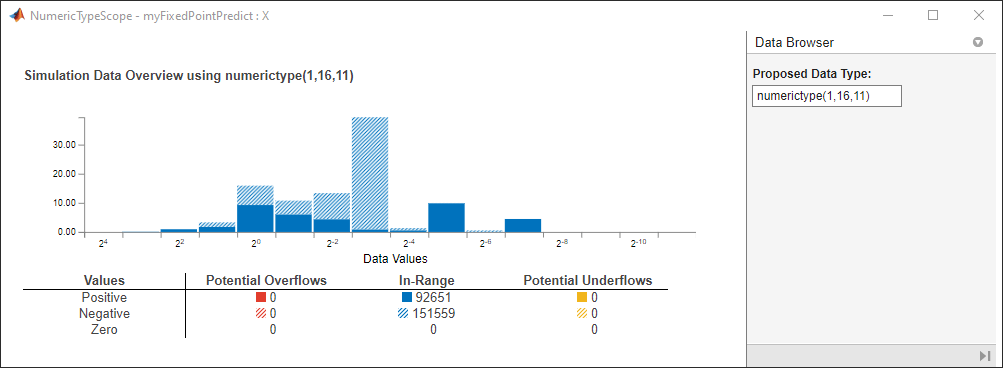The window contains the histogram and dialog panels with information about the variable. For information on this window, see the NumericTypeScope (Fixed-Point Designer) reference page.

Clear the results by using clearInstrumentationResults (Fixed-Point Designer).

clearInstrumentationResults('myFixedPointPredict_instrumented')

Verify Instrumented MEX Function

Compare the outputs from predict and myFixedPointPredict_instrumented.

[labels,scores] = predict(Mdl,standardizedX);
verify_labels1 = isequal(labels,labels_fx1)
verify_labels1 = logical
0

isequal returns logical 1 (true) if labels and labels_fx1 are equal. If the labels are not equal, you can compute the percentage of incorrectly classified labels as follows.

diff_labels1 = sum(strcmp(string(labels_fx1),string(labels))==0)/length(labels_fx1)*100
diff_labels1 = 0.1228

Find the maximum of the relative differences between the score outputs.

diff_scores1 = max(abs((scores_fx1.double(:,1)-scores(:,1))./scores(:,1)))
diff_scores1 = 58.6019

Tune Fixed-Point Data Types

You can tune the fixed-point data types if the recorded results show overflow or underflow, or if you want to improve the precision of the generated code. Modify the fixed-point data types by updating the myMdl_datatype function and creating a new structure, and then generate the code using the new structure. To update the myMdl_datatype function, you can manually modify the fixed-point data types in the function file (myMdl_datatype.m). Or, you can generate the function by using generateLearnerDataTypeFcn and specifying a longer word length, as shown in this example. For more details, see Tips.

Generate a new data type function. Specify the word length 32 and the name myMdl_datatype2 for the generated function.

generateLearnerDataTypeFcn('myMdl',[standardizedX; standardizedXTest],'WordLength',32,'OutputFunctionName','myMdl_datatype2')

Display the contents of myMdl_datatype2.m.

type myMdl_datatype2.m
function T = myMdl_datatype2(dt)
%MYMDL_DATATYPE2 Define data types for fixed-point code generation
%
%   T = MYMDL_DATATYPE2(DT) returns the data type structure T, which defines
%   data types for the variables required to generate fixed-point C/C++ code
%   for prediction of a machine learning model. Each field of T contains a
%   fixed-point object returned by fi. The input argument dt specifies the
%   DataType property of the fixed-point object. Specify dt as 'Fixed' (default)
%   for fixed-point code generation or specify dt as 'Double' to simulate
%   floating-point behavior of the fixed-point code.
%
%   Use the output structure T as both an input argument of an entry-point
%   function and the second input argument of loadLearnerForCoder within the

%   File: myMdl_datatype2.m
%   Statistics and Machine Learning Toolbox Version 12.2 (Release R2021b)
%   Generated by MATLAB, 01-Sep-2021 14:33:50

if nargin < 1
dt = 'Fixed';
end

% Set fixed-point math settings
fm = fimath('RoundingMethod','Floor', ...
'OverflowAction','Wrap', ...
'ProductMode','FullPrecision', ...
'MaxProductWordLength',128, ...
'SumMode','FullPrecision', ...
'MaxSumWordLength',128);

% Data type for predictor data
T.XDataType = fi([],true,32,27,fm,'DataType',dt);

% Data type for output score
T.ScoreDataType = fi([],true,32,30,fm,'DataType',dt);

% Internal variables
% Data type of the squared distance dist = (x-sv)^2 for the Gaussian kernel G(x,sv) = exp(-dist),
% where x is the predictor data for an observation and sv is a support vector
T.InnerProductDataType = fi([],true,32,22,fm,'DataType',dt);

end

The myMdl_datatype2 function specifies the word length 32 and proposes the maximum fraction length to avoid overflows.

Create a structure T2 that defines the fixed-point data types by using myMdl_datatype2.

T2 = myMdl_datatype2('Fixed')
T2 = struct with fields:
XDataType: [0x0 embedded.fi]
ScoreDataType: [0x0 embedded.fi]
InnerProductDataType: [0x0 embedded.fi]

Create a new instrumented MEX function, record the results, and view the results by using buildInstrumentedMex and showInstrumentationResults.

X_fx2 = cast(standardizedX,'like',T2.XDataType);
buildInstrumentedMex myFixedPointPredict -args {X_fx2,coder.Constant(T2)} -o myFixedPointPredict_instrumented2 -histogram -coder
Code generation successful.
[labels_fx2,scores_fx2] = myFixedPointPredict_instrumented2(X_fx2,T2);
showInstrumentationResults('myFixedPointPredict_instrumented2')

Review the instrumentation report, and then clear the results.

clearInstrumentationResults('myFixedPointPredict_instrumented2')

Verify myFixedPointPredict_instrumented2.

verify_labels2 = isequal(labels,labels_fx2)
verify_labels2 = logical
0

diff_labels2 = sum(strcmp(string(labels_fx2),string(labels))==0)/length(labels_fx2)*100
diff_labels2 = 0.0031
diff_scores2 = max(abs((scores_fx2.double(:,1)-scores(:,1))./scores(:,1)))
diff_scores2 = 2.7971

The percentage of incorrectly classified labels diff_labels2 and the relative difference in score values diff_scores2 are smaller than those from the previous MEX function generated using the default word length (16).

For more details about optimizing fixed-point data types by instrumenting MATLAB® code, see the reference pages buildInstrumentedMex (Fixed-Point Designer), showInstrumentationResults (Fixed-Point Designer), and clearInstrumentationResults (Fixed-Point Designer), and the example Set Data Types Using Min/Max Instrumentation (Fixed-Point Designer).

Generate Code

Generate code for the entry-point function using codegen. Instead of specifying a variable-size input for a predictor data set, specify a fixed-size input by using coder.typeof. If you know the size of the predictor data set that you pass to the generated code, then generating code for a fixed-size input is preferable for the simplicity of the code.

codegen myFixedPointPredict -args {coder.typeof(X_fx2,[1,5],[0,0]),coder.Constant(T2)}
Code generation successful.

codegen generates the MEX function myFixedPointPredict_mex with a platform-dependent extension.

Verify Generated Code

You can verify the myFixedPointPredict_mex function in the same way that you verify the instrumented MEX function. See the Verify Instrumented MEX Function section for details.

[labels_sampled,scores_sampled] = predict(Mdl,standardizedX_sampled);
n = size(standardizedX_sampled,1);
labels_fx = true(n,1);
scores_fx = zeros(n,2);
for i = 1:n
[labels_fx(i),scores_fx(i,:)] = myFixedPointPredict_mex(X_fx2(idx(i),:),T2);
end
verify_labels = isequal(labels_sampled,labels_fx)
verify_labels = logical
1

diff_labels = sum(strcmp(string(labels_fx),string(labels_sampled))==0)/length(labels_fx)*100
diff_labels = 0
diff_scores = max(abs((scores_fx(:,1)-scores_sampled(:,1))./scores_sampled(:,1)))
diff_scores = 0.0642

Memory Use

A good practice is to manually standardize predictor data before training a model. If you use the 'Standardize' name-value pair argument instead, then the generated fixed-point code includes standardization operations, which can cause loss of precision and increased memory use.

If you generate a static library, you can find the memory use of the generated code by using a code generation report. Specify -config:lib to generate a static library, and use the -report option to generate a code generation report.

codegen myFixedPointPredict -args {coder.typeof(X_fx2,[1,5],[0,0]),coder.Constant(T2)} -o myFixedPointPredict_lib -config:lib -report

On the Summary tab of the code generation report, click Code Metrics. The Function Information section shows the accumulated stack size.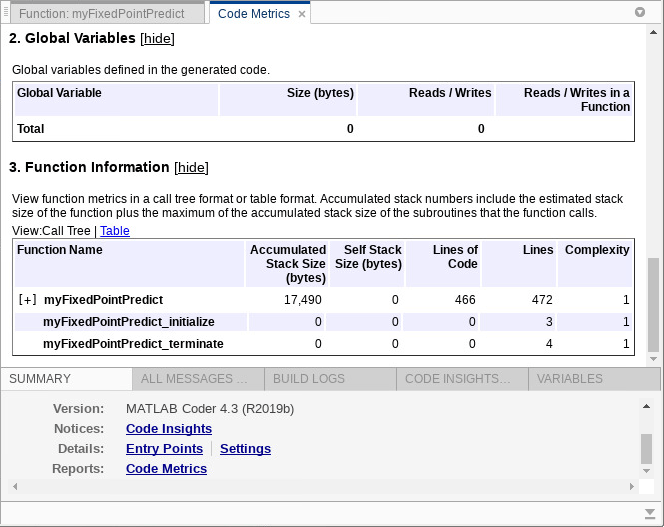To find the memory use of a model trained with 'Standardized','true', you can run the following code.

Mdl = fitcsvm(X_sampled,Y_sampled,'Weight',w_sampled,'KernelFunction','gaussian','Standardize',true);
saveLearnerForCoder(Mdl,'myMdl');
generateLearnerDataTypeFcn('myMdl',[X; XTest],'WordLength',32,'OutputFunctionName','myMdl_standardize_datatype')
T3 = myMdl_standardize_datatype('Fixed');
X_fx3 = cast(X_sampled,'like',T3.XDataType);
codegen myFixedPointPredict -args {coder.typeof(X_fx3,[1,5],[0,0]),coder.Constant(T3)} -o myFixedPointPredict_standardize_lib -config:lib -report